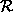Next: Propagation Up: Declarative Modelling and Efficient Previous: Constraints for Multi-Directional Programming

### Constraint Logic programming

Soon an even more radical step was taken when it was recognised that unification could be replaced by any constraint system and solver, provided certain conditions were satisfied. There was no need for a unification algorithm (which reduces an equation between expressions to an equivalent set of variable assignments). Indeed the constraints need not be equations at all.

The resulting scheme

(Jaffar and Lassez, 1987) called the Constraint Logic Programming Scheme, and written CLP(X), was illustrated by choosing mathematical equations and inequations as the constraint system, and the Simplex algorithm as the solver. This instance of CLP(X) is called CLP(), and is described in a later section.

It has inspired a whole research area, exploring the interface between logic and mathematical programming. One resulting constraint programming language is 2LP (Linear Programming and Logic Programming) which embeds mixed integer programming in a constraint programming system. Another is Newton, which uses interval constraints to do solve hard mathematical problems involving polynomials.

Whilst constraint logic programming offers a powerful modelling language, new constraint propagation algorithms and a clean execution model, mathematical programming offers some sophisticated algorithms, highly optimised implementations and a wealth of industrial application know-how.

The next step beyond the standard constraint logic programming scheme was to include more than one constraint system and solver in a single system. Even CLP() was, in fact, such a combination including syntactic unification, Gaussian elimination and the Simplex. CLP systems nowadays include a variety of solvers which exchange information through shared variables. For numeric variables, in addition to the above solvers, there may also be a Groebner base rewriting system for handling polynomial equations, a very powerful CAD solver and a weaker but very useful constraint handler for reasoning on numeric intervals. The latter three system are typically useful for non-linear constraints, containing expressions in which variables are multiplied together.Next: Propagation Up: Declarative Modelling and Efficient Previous: Constraints for Multi-Directional Programming

Mark Wallace
Wed Sep 3 18:36:40 BST 1997